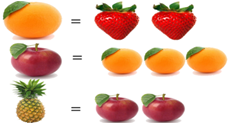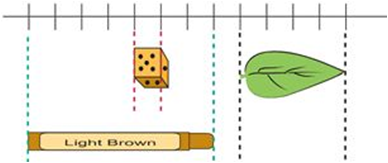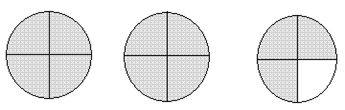SilverZone - IOM PDF Sample Papers for Class 3

Resources:

Class 3 sample paper & practice questions for International Olympiad of Mathematics (IOM) level 1 are given below. Syllabus for level 1 is also mentioned for these exams. You can refer these sample paper & quiz for preparing for the exam.Sample Questions from Olympiad Success:

 Q.1 Q.2 Q.3 Q.4 Q.5 Q.6 Q.7 Q.8 Q.9 Q.10
 Q.1 If each strawberry weighs 37 g, then find the weight of pineapple according to the relation given in the image:a) 404 g b) 454 g c) 424 g d) 444 g
 Q.2 I am a two-digit number. If 28 is added to me, I become a three-digit number and if 63 is subtracted from me, I become one-digit number. Which number I am? a) 56 b) 69 c) 72 d) 98
 Q.3 Choose the correct option: The die is 1 cm from every side, then  the leaf is _________ cm long.a) 10 cm b) 4 cm c) 6 cm d) 8 cm
 Q.4 Which of the following options has the smallest value? a) 4 + 3 + 2 + 1 b) 4 + 3 + 2 x 1 c) 4 + 3 x 2 + 1 d) 4 x 3 + 2 + 1
 Q.5 How many sevens are there in 735? (IMO L2 2012) a) 15 b) 105 c) 17 d) 150
 Q.6 What fraction is the shaded part?a) 2 b) 2 1/4 c) 1 3/4 d) 2 3/4
 Q.7 Anu went to watch a movie. She bought a movie ticket for Rs. 180, 3 packets of popcorn for Rs. 60, and 2 cold drinks worth Rs. 25 each. What is the total money that she had spent on the movie? a) Rs. 385 b) Rs. 265 c) Rs. 290 d) Rs. 410
 Q.8 Arrange these numbers from the greatest to the smallest:56, 3 × 8, 29, 2 × 7, 2, 19.   (IMO L2 2012) a) 56, 2 × 7, 3 × 8, 29, 2, 19. b) 2, 2 × 7, 19, 3 × 8, 29, 56. c) 56, 29, 3 × 8, 19, 2 × 7, 2. d) 2 × 7, 3 × 8, 29, 2, 19, 56.
 Q.9 Sam bought one kg of tea in a plastic jar. The jar is filled to the brim and could not contain any more tea. Choose the correct statement:  ( IMO L2 2017) a) The capacity of the plastic jar is 10 hg of tea. b) The capacity of the plastic jar is 1,000 g of tea. c) The capacity of the plastic jar is 1,000,000 mg of tea. d) All of these.
 Q.10 Rahul has five notes of rupees twenty, six notes of rupees ten, three notes of rupees five and two notes of rupees fifty. If he bought a toy of rupees two hundred and eighteen, then how much money does he have now? a) Rs. 57 b) Rs. 55 c) Rs. 59 d) Rs. 47Sample PDF of SilverZone - International Olympiad of Mathematics (IOM) PDF Sample Papers for Class 3: